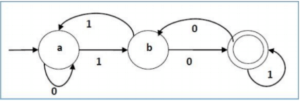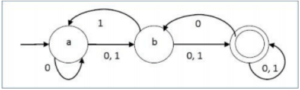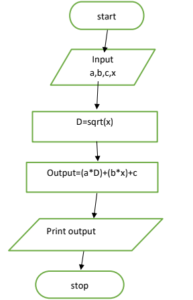# Formal Language and Automata Theory (FLAT)

## Define Automata and its type with a suitable diagram?

The term “Automaton “(plural automata) is derived from the  Greek word “αὐτόματα,” which means “self-acting”. Automata is the abstract model of a digital computer. It has a mechanism of reading input which is written on an input file to which the automata can read but not change.

It follows a predetermined sequence of operations automatically.

Types of Automata:
1. Deterministic Automata
2. Non-Deterministic Automata

Deterministic Automata: A deterministic automaton is a concept of automata theory in which the outcome of a transition from one state to another is determined by the input. A common deterministic automaton is a DFA (deterministic finite automaton).Non-Deterministic Automata: In non-deterministic automata, it is not defining the move of automata at each point. An NDA may have several possible moves. So, we can only predict a set of possible actions, not move. A common non-deterministic automaton is an NFA(non-deterministic finite automata).## Define the Algorithm and its types?

An algorithm is a finite set of rules which gives a sequence of operations of solving a specific problem. The structure of an algorithm can be defined as:

• The input step
• The assignment step
• The decision step
• The repetitive step
•  The output step

Types of an algorithm:

1. Deterministic algorithm: After the execution of each step of an algorithm. The control logic comes to the decision box with two paths one for yes and one for no.

2. Non-Deterministic algorithm: If the algorithm is capable of exploring a large number of alternatives simultaneously to reach out to a correct solution. We can call it NDA. E.g.: Race  Condition.

3. Random algorithm: If after executing some step the control logic transfer to another step of the algorithm as dictated by the random device. E.g.: coin tossing.

4. Infinite algorithm: An algorithm whose loop is running continues to give us better and better estimates of the results. e.g.: digital clock.

## Define the flow chart and draw a flowchart to find all the roots of a quadratic equation

### ax2+bx+c=0.

A flow chart is a graphical representation of a specific sequence of steps of an algorithm.  It consists of a diagram of characteristically shaped boxes connected by directed line segments.

Flowchart to calculate roots of quadratic equation ax2+bx+c=0.## Define the Term?

### Alphabet in Automata

Ans – An alphabet can be defined as a finite set of symbols. These are the input symbols from which strings are constructed by Appling certain operation in automata theory we denote alphabet or input symbol by the set ∑. e.g.: ∑ ={0,1}.

### String in automata

Ans Combine some symbols with each other by applying some rules we get a string. The string can be also obtained by applying operation on a particular symbol itself. E.g.: { abc}.

### Language in automata

Ans – The alphabet is a set of string from a  language while using some criteria. E.g. let us consider the set of all-natural number N or set of even number etc.

Grammar in automata
Ans –
The language of grammar is the set of all string that can be generated from that grammar.